# Dividing a Circle

#### To practice this technique you will need a pencil, paper, a compass, and a ruler.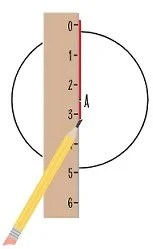STEP 1 Lay a rule across the circle so that it touches center point A that was created by the point of the compass. STEP 2 Pull a line across the circle with your pencil.  This will automatically divide the circle into two equal parts.  Mark the points where the line intersects the circle as B and C.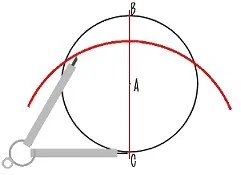STEP 3 Place the point of the compass at point C.  Open the compass so that it’s angle is greater than one half the circle. STEP 4 Draw a arc across the circle. Allow it to extend well beyond the circle’s edges.STEP 5 Using the exact same opening on the compass, repeat Step 4 placing the compass point at B.  Note where the two arcs intersect. STEP 6 Lay a ruler across the circle so that it touches the arc intersections and the center point. Draw a pencil line.STEP 7 The second line you have created is automatically perpendicular to the original line, lying at a 90 degree angle to the first. STEP 8 Mark where the second line intersects the circle as points D and E. You can now divide the pie slice of the circle (shown in pink) by repeating steps 4 through 7 and using points D and B.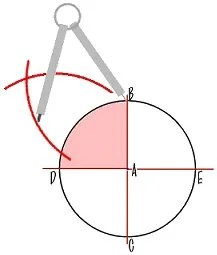STEP 9 Again open the compass wider than one half the area to be divided.  Place the point of the compass at D and pull an arc.  Move the compass, don’t change the compass opening,  to point B and pull a second arc. STEP 10 Place the ruler at the intersection of the two arcs and so that it touches the center A.  Pull a line with your pencil all the way through the circle.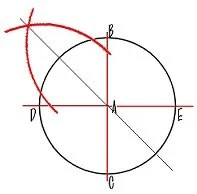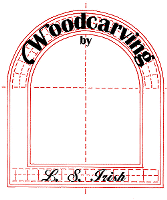STEP 11 Dividing one side of the circle will automatically give you the correct line for the opposite pie slice in the circle. STEP 12 Each time you draw a line you create a new slice of the pie.  You can continue to work from the points on the circle for each of these slice and divide the circle as many times as you need. You can see in the lettering pattern above how this technique is used in wood carving. Lettering Layouts for Wood Carving